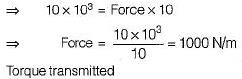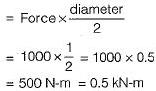# Gears - Mechanical Engineering - 2

## 10 Questions MCQ Test Topicwise Question Bank for GATE Mechanical Engineering | Gears - Mechanical Engineering - 2

Description
This mock test of Gears - Mechanical Engineering - 2 for Mechanical Engineering helps you for every Mechanical Engineering entrance exam. This contains 10 Multiple Choice Questions for Mechanical Engineering Gears - Mechanical Engineering - 2 (mcq) to study with solutions a complete question bank. The solved questions answers in this Gears - Mechanical Engineering - 2 quiz give you a good mix of easy questions and tough questions. Mechanical Engineering students definitely take this Gears - Mechanical Engineering - 2 exercise for a better result in the exam. You can find other Gears - Mechanical Engineering - 2 extra questions, long questions & short questions for Mechanical Engineering on EduRev as well by searching above.
QUESTION: 1

Solution:
QUESTION: 2

Solution:
QUESTION: 3

### Select the odd terminology in respect of bevel gears:

Solution: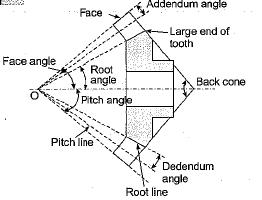QUESTION: 4

The role of hunting tooth or hunting cog in a gear transmission is that it

Solution:

There is concept of ‘hunting tooth’ for uniform distribution of tooth wear. Suppose (ZP = 20), then after every two revolutions of the pinion, the same pair of teeth will engage. If however, we take (ZP = 20) and (Zg = 4), the pinion will rotate 41 times before the same pair of teeth will engage again. This extra teeth is called the hunting tooth. It results in more even distribution of wear.

QUESTION: 5

Lewis form factor (y) depends upon

Solution:

Lewis form factor for 200 full depth involute system is give by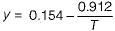Where T = Number of teeth.
Hence the value of y is depends only on the number of teeth and it is independent on size of the tooth.

QUESTION: 6

A triple threaded worm has teeth of 6 mm module and pitch circle diameter of 50 mm. If the worm gear has 20 teeth of 200 and the coefficient of friction of the worm gearing is 0.05. The lead angle of worm is

Solution:

m = 6, n = 3 mm Dw = 50 mm lead angle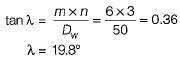QUESTION: 7

In the formulation of lewis equation for toothed gearing. It is assumed that tangential tooth load FT Exacts of the

Solution:
QUESTION: 8

The dynamic load on a gear is due to:
1. Inaccuracies of tooth spacing.
2. Irregularities in tooth profile.
3. Deflection of the teeth under load.
4. Type of service (i.e., intermittent, one shift per day, continuous per day).
Which of the above statements are correct?

Solution:

The dynamic load between the meshing teeth arises due to following factors:
1. Inaccuracies of tooth profile
2. Error in tooth spacing
3. Runout of gear
4. Gear mesh stiffness variation
5. Inertia of rotating masses
6. Deflection of teeth
7. Stiffness of rotating parts
But dynamic factor mainly depends upon tooth error and pitch line velocity.

QUESTION: 9

Large speed reductions (greater than 20) in one stage of a gear train are possible through

Solution:
QUESTION: 10

A spur gear transmits 10 kW at a pitch line velocity of 10 m/s; driving gear has a diameter of 1.0 m. Find the tangential force between the driver and the follower, and the transmitted torque respectively.

Solution:

Power transmitted = Force x Velocity